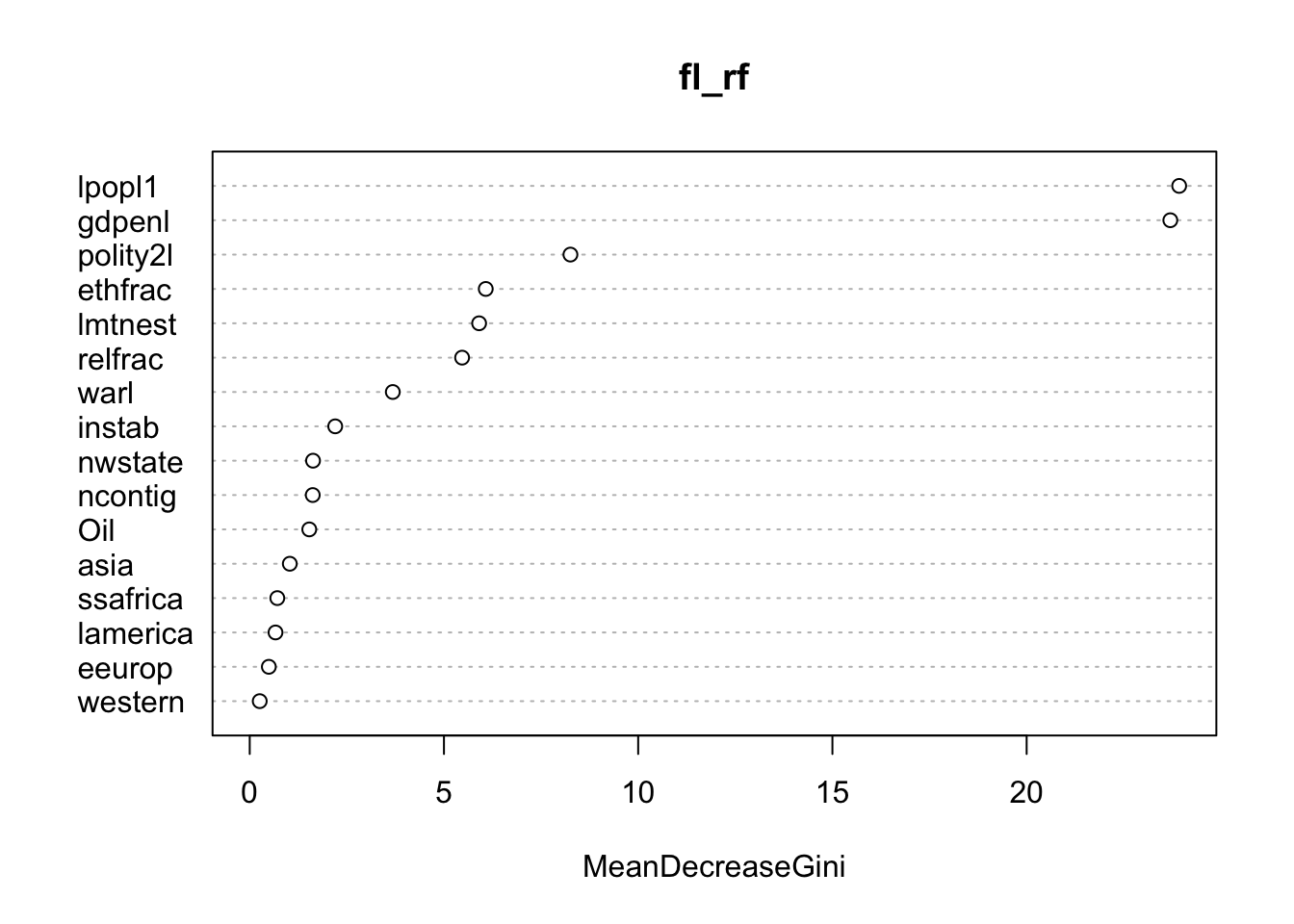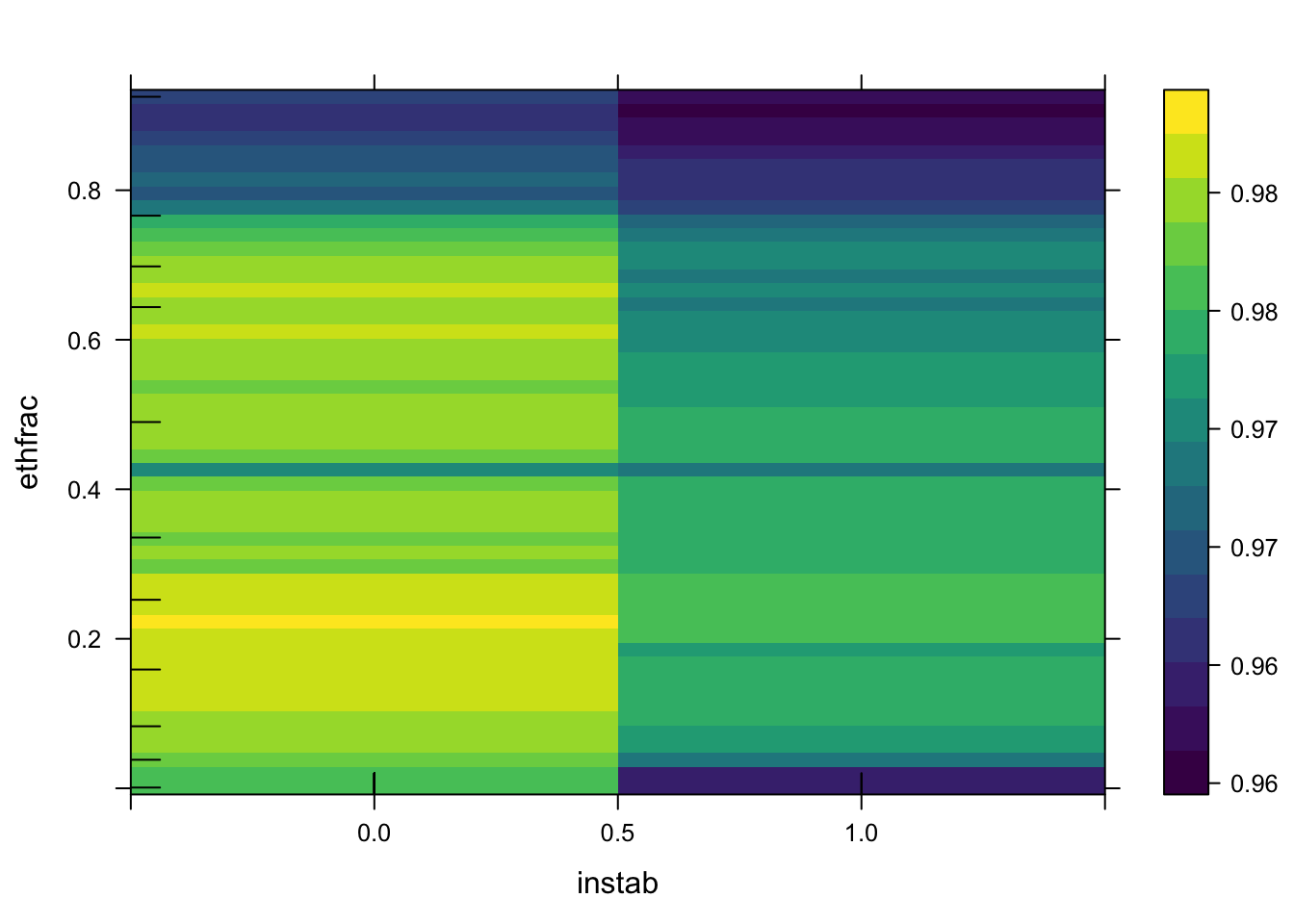## Individual Exercise Solution

In addition to regression trees, we can also fit classification trees when we have binary or categorical outcomes. Use `fl2003.RData`, which is a subset of the data in Fearon and Laitin (2003), to fit an ensemble model that explains `onset` as a function of all other variables. Determine the most important variables in the ensemble, and then produce a partial dependence plot showing the relationship between two variables that are not the most important, and the predicted probability of civil war in a given observation. Discuss this relationship.

``````# set seed for replication
set.seed(0032185)

library(randomForest) # random forest ensembles
library(pdp) # partial dependence plots
library(doParallel) # parallel processing

# register parallel backend
registerDoParallel(makeCluster(parallel::detectCores()))

# split into training and test sets
train <- sample(1:nrow(fl), (2 / 3) * nrow(fl))
fl_train <- fl[train, ]
fl_test <- fl[-train, ]

# fit random forest model
fl_rf <- randomForest(formula = as.factor(onset) ~., data = fl_train,
ntree = 1500, mtry = 3, nodesize = 1)

# variable importance plot
varImpPlot(fl_rf)````````````# partial dependence
fl_part <- partial(fl_rf, pred.var = c('instab', 'ethfrac'), rug = T,
train = fl_train, which.class = 1, prob = T, parallel = T,
paropts = list(.packages = "randomForest"))

# 2D plot
plotPartial(fl_part, rug = T, train = fl_train)````````````# 3D plot
plotPartial(fl_part, train = fl_train, levelplot = F, drape = T, colorkey = T,
screen = list(z = 240, x = -60))``````